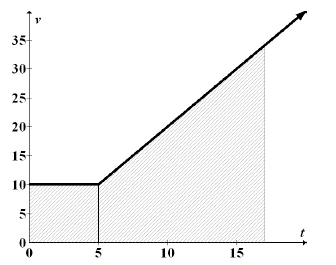### Home > PC > Chapter 5 > Lesson 5.2.5 > Problem5-103

5-103.

The velocity of a car (in feet per second) is given by $v ( t ) = \left\{ \begin{array} { l l } { 10 } & { \text { for } 0 < t \leq 5 } \\ { 2 t } & { \text { for } t > 5 } \end{array} \right.$. How long does it take for the car to travel a total of $250$ft?

1. Graph.
2. Distance is area under a velocity curve.
3. When will the area under the curve $= 250$ ft?

$\frac{\text{feet}}{\text{second}}$seconds